# JP6346850B2 - Modeling apparatus, modeling method, and modeling program - Google Patents

## Info

Publication number
JP6346850B2
JP6346850B2 JP2014233878A JP2014233878A JP6346850B2 JP 6346850 B2 JP6346850 B2 JP 6346850B2 JP 2014233878 A JP2014233878 A JP 2014233878A JP 2014233878 A JP2014233878 A JP 2014233878A JP 6346850 B2 JP6346850 B2 JP 6346850B2
Authority
JP
Japan
Prior art keywords
point
function
set
points
plurality
Prior art date
Legal status (The legal status is an assumption and is not a legal conclusion. Google has not performed a legal analysis and makes no representation as to the accuracy of the status listed.)
Active
Application number
JP2014233878A
Other languages
Japanese (ja)
Other versions
JP2016096854A (en
Inventor

Original Assignee

Priority date (The priority date is an assumption and is not a legal conclusion. Google has not performed a legal analysis and makes no representation as to the accuracy of the date listed.)
Filing date
Publication date
Application filed by 富士通株式会社, 国立大学法人 東京大学, 国立大学法人 東京大学 filed Critical 富士通株式会社
Priority to JP2014233878A priority Critical patent/JP6346850B2/en
Publication of JP2016096854A publication Critical patent/JP2016096854A/en
Application granted granted Critical
Publication of JP6346850B2 publication Critical patent/JP6346850B2/en
Active legal-status Critical Current
Anticipated expiration legal-status Critical

• 238000004364 calculation methods Methods 0 claims description 70
• 238000000034 methods Methods 0 description 46
• 210000001765 Aortic Valve Anatomy 0 description 2
• 210000004115 Mitral Valve Anatomy 0 description 2

## Images

•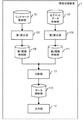••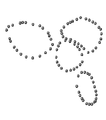•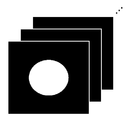•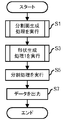•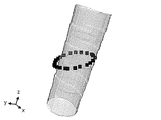•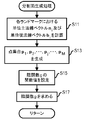••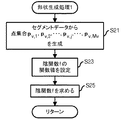••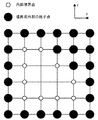•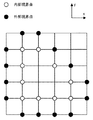••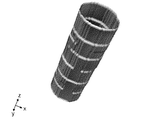•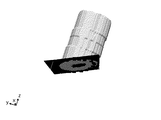•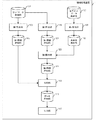•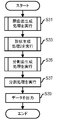••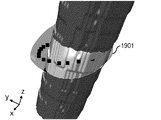•••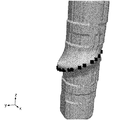•••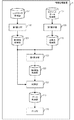•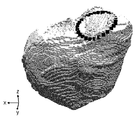•••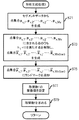•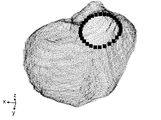•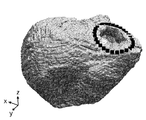•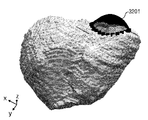••## Classifications

• GPHYSICS
• G06COMPUTING; CALCULATING; COUNTING
• G06TIMAGE DATA PROCESSING OR GENERATION, IN GENERAL
• G06T7/00Image analysis
• G06T7/60Analysis of geometric attributes
• GPHYSICS
• G16INFORMATION AND COMMUNICATION TECHNOLOGY [ICT] SPECIALLY ADAPTED FOR SPECIFIC APPLICATION FIELDS
• G16HHEALTHCARE INFORMATICS, i.e. INFORMATION AND COMMUNICATION TECHNOLOGY [ICT] SPECIALLY ADAPTED FOR THE HANDLING OR PROCESSING OF MEDICAL OR HEALTHCARE DATA
• G16H50/00ICT specially adapted for medical diagnosis, medical simulation or medical data mining; ICT specially adapted for detecting, monitoring or modelling epidemics or pandemics
• G16H50/50ICT specially adapted for medical diagnosis, medical simulation or medical data mining; ICT specially adapted for detecting, monitoring or modelling epidemics or pandemics for simulation or modelling of medical disorders

## Description

The present invention relates to a modeling apparatus, a modeling method, and a modeling program technique.

Development of an organ simulator for simulating organ dynamics is underway, and the organ simulator is expected to be useful for diagnosis, determination of treatment policy, and postoperative prediction. In order to increase the reliability of the simulator, it is preferable that the shape of the organ used for the simulation is as close as possible to the actual shape of the organ.

Since the shape of the organ differs from patient to patient and the same shape cannot be used uniformly, the shape of the organ is generated for each patient. However, since the organ exists inside the living body and cannot be visually observed or directly measured, the organ is generated based on a medical image such as CT (Computed Tomography) or MRI (Magnetic Resonance Imaging) of the patient. A certain document discloses a technique for generating a shape of a patient's organ by deforming a template representing a standard shape of the organ based on landmarks arranged on the medical image of the patient.

However, there is no known technique for generating a shape by paying attention to an annular structure in an organ. For example, the heart has a pulmonary valve, aortic valve, mitral valve, and tricuspid valve, and the edge portion of each valve is called an annulus. Since the valve plays an important role in the function of the heart as a pump, generating the shape near the annulus with high accuracy leads to an increase in the reliability of the simulator.

JP 2013-015945 A

"A Multi-scale Approach to 3D Scattered Data Interpolation with Compactly Supported Basis Functions", Yutaka Ohtake, Alexander Belyaev, and Hans-Peter Seidel, Shape Modeling International 2003, Seoul, Korea, May 2003, pp153-161

Therefore, an object of the present invention is, in one aspect, to provide a technique for generating a shape of an object including an annular structure with high accuracy.

A modeling device according to the present invention includes a first calculation unit that calculates a first function for generating a closed curved surface including a plurality of points based on position information of a plurality of points representing an annular structure in an object; A first generation unit that generates a first point set representing a boundary of an object from a plurality of tomographic images each of which an area occupied by the object is specified, and a point that exists inside the closed curved surface of the first point set A second point set is generated by detecting using the first function and deleting the detected points from the first point set, and using the second point set and the plurality of points, And a second calculation unit that calculates a second function for generating the shape.

In one aspect, the shape of an object including an annular structure can be generated with high accuracy.

FIG. 1 is a functional block diagram of the information processing apparatus according to the first embodiment. FIG. 2 is a diagram for explaining landmarks. FIG. 3 is a diagram for explaining landmarks. FIG. 4 is a diagram for explaining segment data. FIG. 5 is a diagram showing a main processing flow in the first embodiment. FIG. 6 is a diagram showing objects and landmarks handled in the first embodiment. FIG. 7 is a diagram illustrating a process flow of the split surface generation process. FIG. 8 is a diagram for explaining the dividing plane. FIG. 9 is a diagram illustrating a process flow of the shape generation process in the first embodiment. FIG. 10 is a diagram for explaining the internal boundary points and the external boundary points. FIG. 11 is a diagram for explaining the internal boundary points and the external boundary points. FIG. 12 is a diagram for explaining the internal boundary points and the external boundary points. FIG. 13 is a diagram for explaining the internal boundary points and the external boundary points. FIG. 14 is a diagram illustrating the boundary of an object. FIG. 15 is a diagram illustrating the shape of an object divided by the dividing surface. FIG. 16 is a functional block diagram of the information processing apparatus according to the second embodiment. FIG. 17 is a diagram illustrating a main processing flow in the second embodiment. FIG. 18 is a diagram illustrating a processing flow of closed curved surface generation processing. FIG. 19 is a diagram for explaining a closed curved surface. FIG. 20 is a diagram illustrating a processing flow of the shape generation processing in the second embodiment. FIG. 21 is a diagram illustrating a point set representing an object boundary. FIG. 22 is a diagram illustrating the boundary of an object. FIG. 23 is a diagram for explaining the dividing plane. FIG. 24 is a diagram illustrating the shape of the object divided by the dividing surface. FIG. 25 is a functional block diagram of the information processing apparatus according to the third embodiment. FIG. 26 is a diagram illustrating landmarks arranged at the position of the annulus. FIG. 27 is a diagram illustrating a main processing flow in the third embodiment. FIG. 28 is a diagram for explaining a closed curved surface generated by the implicit function h 1 . FIG. 29 is a diagram illustrating a processing flow of the shape generation processing in the third embodiment. FIG. 30 is a diagram illustrating a point set representing a ventricular boundary. FIG. 31 is a diagram showing a corrected shape. FIG. 32 is a diagram for explaining a closed curved surface generated by the implicit function h 2 . FIG. 33 is a diagram showing a corrected shape. FIG. 34 is a functional block diagram of a computer.

[Embodiment 1]
In the first embodiment, an example in which the shape of an object is divided by a curved surface passing through landmarks arranged in an annular shape will be described.

FIG. 1 shows a functional block diagram of the information processing apparatus 1 according to the first embodiment. The information processing apparatus 1 includes a landmark storage unit 101, a first calculation unit 103, a first function storage unit 105, a segment data storage unit 107, a second calculation unit 109, a second function storage unit 111, A dividing unit 113, a data storage unit 115, and an output unit 117 are included.

The first calculation unit 103 executes processing based on the data stored in the landmark storage unit 101 and stores the processing result in the first function storage unit 105. The second calculation unit 109 executes processing based on the data stored in the segment data storage unit 107 and stores the processing result in the second function storage unit 111. The dividing unit 113 executes processing based on the data stored in the first function storage unit 105 and the data stored in the second function storage unit 111 and stores the processing result in the data storage unit 115. The output unit 117 outputs the data stored in the data storage unit 115.

FIG. 2 shows an example of landmarks arranged on the medical image. FIG. 2 is a view showing a medical image of the ventricle, and landmarks 201 to 204 are arranged on two annulus. The cutting plane is substantially orthogonal to the plane passing through the annulus. The radiogram interpreter arranges landmarks as shown in FIG. 2 for a plurality of layers of medical images. Then, when landmarks arranged for a plurality of layers of medical images are extracted, for example, as shown in FIG. In the example of FIG. 3, landmarks arranged for each of the pulmonary valve, aortic valve, mitral valve, and tricuspid valve are shown. The landmark storage unit 101 stores position information of the arranged landmarks (for example, information indicating the position on the three-dimensional structured grid).

The segment data stored in the segment data storage unit 107 is three-dimensional structured grid data. The three-dimensional structured grid is a rectangular parallelepiped uniform grid. A label value “1” is assigned to a lattice point located inside the boundary surface of the object, and a label value “0” is assigned to a lattice point located outside the boundary surface of the object. The segment data is generated from a medical image as shown in FIG. 4 in which a region inside the boundary surface of the object and a region outside the boundary surface of the object are designated. In the example of FIG. 4, the area inside the boundary surface of the object is represented in white, and the area outside the boundary surface of the object is represented in black. The designation of the area is also called segmentation, and the designation is performed by the image reader.

Next, processing executed by the information processing apparatus 1 according to the first embodiment will be described with reference to FIGS. In order to simplify the description, in the first embodiment, an object and landmark as shown in FIG. 6 will be described as an example. In FIG. 6, square figures represent landmarks, and the landmarks are arranged in a ring shape. The object has a cylindrical shape, and the major axis direction of the object coincides with the z-axis direction.

First, the first calculation unit 103 of the information processing apparatus 1 executes a split surface generation process (FIG. 5: Step S1). The split surface generation process will be described with reference to FIGS.

The first calculation unit 103 is based on the position information of the landmarks x 1 , x 2 ,..., X i , x N stored in the landmark storage unit 101, and the unit principal method for each landmark. The line vector n i and the unit normal line vector b i are calculated (FIG. 7: step S11). Here, N is the number of landmarks.

Details of the calculation in step S1 are shown below. First, x cl is calculated as follows.

Next, s i is calculated as follows based on x i .

Next, n i tilde is calculated based on s i as follows.

This calculation is performed to align the direction of the main normal. Next, calculate the n i hat as follows based on the n i tilde.

This calculation is performed to suppress the vibration of the main normal vector. Next, unit main basis of the n i hat the normal vector n i is calculated as follows.

Then, the unit binormal vector b i on the basis of the unit main normal vector n i is calculated as follows.

The first calculation unit 103, the point set p 1, p 2, ···, p j, ···, to produce a p M (step S13). In step S13, the following point set is generated with M = 3N.

That is, the generated point set includes a landmark, a point where the landmark is moved by ε b in the positive direction of the normal line, and a point where the landmark is moved by ε b in the negative direction of the normal line. ε b is a constant, and a value sufficiently smaller than the distance between landmarks is adopted. In the present embodiment, ε b = 1.0.

The first calculation unit 103 sets a function value of the implicit function g (step S15). Specifically, the function value is set as follows. Thereby, it is possible to suppress the occurrence of unevenness on the surface formed by the set of points where the value of the implicit function g is zero.

The first calculation unit 103 obtains the implicit function g so that the function value becomes the value set in step S15 (step S17), and stores the data of the implicit function g in the first function storage unit 105. Then, the process returns to the calling process.

In step S17, α j , β 0 , β 1 , β 2 , and β 3 are determined using TPS (Thin Plate Spline) so as to satisfy the following equations. However, the basis function is φ j (x) = − | x−p j |.

Here, Equations (1), (2), and (3) are set as simultaneous linear equations, and α j , β 0 , β 1 , β are obtained by a general solution (for example, Gaussian elimination method or LU decomposition method). 2 and β 3 are determined. Note that x = (x, y, z) and p j = (p j, x , p j, y , p j, z ).

A surface formed by a set of points where the value of the implicit function g is 0 (hereinafter referred to as a divided surface) is as shown in FIG. In FIG. 8, the dividing surface 801 is represented in black. Split surface 801 passes landmark x 1, x 2, ···, x i, ···, the x N. The value of the implicit function g is positive in the positive direction of the binormal line for the landmark, and the value of the implicit function g is negative in the negative direction of the binormal line for the landmark. Here, a part of the dividing surface is shown so that the relationship between the object and the dividing surface can be easily understood.

Returning to the description of FIG. 5, the second calculation unit 109 executes the shape generation process in the first embodiment (step S <b> 3). The shape generation process in the first embodiment will be described with reference to FIGS.

First, the second calculation unit 109 calculates the point set p v, 1 , p v, 2 ,..., P v, j ,..., P v, from the segment data stored in the segment data storage unit 107 . Mv is generated (FIG. 9: Step S21).

In step S21, point sets p v, 1 , p v, 2 ,..., P v, j ,. The attached point v represents that the point set is the boundary of the object.

First, among the lattice points located inside the boundary surface (that is, lattice points having a label value of 0), at least one of the adjacent lattice points is located outside the boundary surface (that is, the label value is 1). Is set as an internal boundary point. Here, the “adjacent grid points” are two points that are 1d x away from the focused grid point in the x direction (d x is the inter-lattice distance in the x-axis direction), and 1 d y from the focused grid point in the y direction. (D y is the inter-lattice distance in the y-axis direction) 2 points that are separated from each other and 2 d that is 1d z away from the noted lattice point in the z-direction (d z is the inter-lattice distance in the z-axis direction) 6 points including points. However, when the lattice points are located at the end of the space, the number of adjacent lattice points is less than 6.

For example, assume that the segment data in a certain xy plane is in a state as shown in FIG. In FIG. 10, lattice points located inside the boundary surface are represented by white circles, and lattice points located outside the boundary surface are represented by black circles. In this case, when the internal boundary point is set, for example, as shown in FIG. In FIG. 11, the internal boundary points are represented by white circles, and the grid points located outside the boundary surface are represented by black circles. However, the white circles representing the internal boundary points are smaller than the white circles representing the grid points located inside the boundary surface.

Next, among the lattice points located outside the boundary surface, a lattice point in which at least one of the adjacent lattice points is an internal boundary point is set as an external boundary point. For example, when the external boundary point is set in the state as shown in FIG. 11, it is as shown in FIG. In FIG. 12, the inner boundary points are represented by white circles, and the outer boundary points are represented by black circles. However, the black circles representing the external boundary points are smaller than the black circles representing the grid points located outside the boundary surface.

Next, the value of the inner boundary point is set to -1, and the value of the outer boundary point is set to +1. For example, when a value is set in the state as shown in FIG. 12, the result is as shown in FIG.

That is, points included in the point set p v, 1 , p v, 2 ,..., P v, j ,..., P v, Mv are internal boundary points or external boundary points.

Returning to the description of FIG. 9, the second calculation unit 109 sets a function value of the implicit function f (step S23). Specifically, the function value is set as follows.

The first calculation unit 103 obtains the implicit function f so that the function value becomes the value set in step S23 (step S25), and stores the data of the implicit function f in the second function storage unit 111. Then, the process returns to the calling process.

In step S23, the implicit function f is obtained using TPS. In addition, when it takes time to obtain the implicit function f by TPS, the implicit function f may be obtained by the method described in Non-Patent Document 1, for example.

A boundary formed by a set of points at which the value of the implicit function f is 0 (hereinafter referred to as a zero isosurface of the implicit function f) is as shown in FIG. In FIG. 14, the value of the implicit function f is negative inside the boundary (that is, inside the cylinder), and the value of the implicit function f is positive outside the boundary (that is, outside the cylinder).

Returning to the description of FIG. 5, the dividing unit 113 performs division processing based on the implicit function g data stored in the first function storage unit 105 and the implicit function f data stored in the second function storage unit 111. (Step S5), and volume data representing the shape after division is stored in the data storage unit 115.

The dividing process is a process of dividing the shape represented by the 0 isosurface of the implicit function f by the 0 isosurface of the implicit function g. For example, a point set satisfying the implicit function f = 0 and the implicit function g> 0 is obtained in the division process, and volume data regarding the point set is stored in the data storage unit 115.

When volume data is generated with the same structured grid as the segment data, first, a structured grid is generated using the number of grids of the segment data, the voxel size, and the origin coordinates. For each grid point, when the grid point satisfies the implicit function g> 0 and the implicit function f> 0, −1 is set, and the grid point sets the implicit function g> 0 and the implicit function f> 0. If not, set +1. Thereby, binary volume data is generated. Further, for example, mesh data can be generated by pasting a mesh to volume data by the marching cube method.

The output unit 117 outputs the volume data stored in the data storage unit 115 to the display device (step S7). When the volume data and the division plane are displayed, for example, as shown in FIG. As shown in FIG. 15, the object is divided by the dividing surface passing through the landmark, and the portion of the object that satisfies the implicit function g> 0 is output.

If the basis function of the implicit function g, the basis function of the implicit function f, α j , β 0 , β 1 , β 2 , β 3 , and the synthesis method of the implicit function g and the implicit function f are output, the user himself / herself will output. The display of FIG. 15 can be reproduced. This has the advantage that the user can freely change the number of grids when outputting volume data. Further, if the number of grids is reduced, the size of the volume data to be stored is reduced.

By executing the processing as described above, the object can be divided at the portion having the annular structure. If this embodiment is applied to the annulus, a boundary surface that divides the ventricle and the other part can be generated, and therefore the process of extracting only the ventricle or only the other part becomes easy. This makes it easy to set parameters for the ventricle and parameters for the other parts separately. In addition, compared to the case where the shape of the ventricle and the shape of the other part are separately generated and combined, the shape of the combined part is close to the actual shape, so the reliability of the simulator is increased.

[Embodiment 2]
In the second embodiment, an example will be described in which the shape of an object is corrected according to landmarks arranged in an annular shape.

FIG. 16 is a functional block diagram of the information processing apparatus 1 according to the second embodiment. The information processing apparatus 1 includes a landmark storage unit 101, a first calculation unit 103, a first function storage unit 105, a segment data storage unit 107, a second calculation unit 109, a point set storage unit 110, a first 3 calculation unit 119, third function storage unit 121, fourth calculation unit 123, fourth function storage unit 125, division unit 113, data storage unit 115, and output unit 117.

The first calculation unit 103 executes processing based on the data stored in the landmark storage unit 101 and stores the processing result in the first function storage unit 105. The second calculation unit 109 executes processing based on the data stored in the segment data storage unit 107 and stores the processing result in the point set storage unit 110. The third calculation unit 119 executes processing based on the data stored in the landmark storage unit 101 and stores the processing result in the third function storage unit 121. The fourth calculation unit 123 executes processing using the data stored in the point set storage unit 110 and the data stored in the third function storage unit 121 and stores the processing result in the fourth function storage unit 125. The dividing unit 113 executes processing based on the data stored in the first function storage unit 105 and the data stored in the fourth function storage unit 125 and stores the processing result in the data storage unit 115. The output unit 117 outputs the data stored in the data storage unit 115.

Next, processing executed by the information processing apparatus 1 according to the second embodiment will be described with reference to FIGS. In the second embodiment, an object and landmark as shown in FIG. 6 will be described as an example.

First, the third calculation unit 119 executes a closed curved surface generation process (FIG. 17: step S31). The closed curved surface generation processing will be described with reference to FIGS.

The third calculation unit 119, the landmark x 1 stored in the landmark storage unit 101, x 2, ···, x i, ···, based on the position information of the x N, unit main methods in each landmark The line vector n i and the unit normal line vector b i are calculated (FIG. 18: step S41). Since this process is the same as step S11 in the first embodiment, a description thereof will be omitted.

The third calculation unit 119, the point set p 1, p 2, ···, p j, ···, to produce a p M (step S43). In step S43, the following point set is generated with M = 5N.

That is, the generated point set includes a point where the landmark is moved D in the positive direction of the normal, a point where the landmark is moved in the positive direction of the normal (D + ε b ), and the landmark is moved in the positive direction of the normal. (D−ε b ), and the landmark moved D in the positive direction of the normal and 7ε b moved in the positive direction of the normal and the landmark moved D in the positive direction of the normal. And a point moved by 7ε b in the negative direction of the normal line. ε b is a constant, and a value sufficiently smaller than the distance between landmarks is adopted. In the present embodiment, ε b = 1.0. D is preferably set so that the closed curved surface includes a landmark and a boundary on the curved surface passing through the landmark. In the present embodiment, and D = 5ε b.

The third calculation unit 119 sets a function value of the implicit function h (step S45). Specifically, the function value is set as follows. Thereby, a point set satisfying h = 0 forms a closed curved surface including landmarks arranged in a ring shape.

The third calculation unit 119 obtains the implicit function h so that the function value becomes the value set in step S45 (step S47), and stores the data of the implicit function h in the third function storage unit 121. Then, the process returns to the calling process. Also in step S47, the implicit function h is obtained using TPS. Since the basic idea is the same as the processing in step S17, the description is omitted.

A surface formed by a set of points where the value of the implicit function h is 0 (hereinafter referred to as the 0 equivalent surface of the implicit function h) is as shown in FIG. In FIG. 19, a square figure represents a landmark, and a zero isosurface 1901 of an implicit function h is a closed curved surface having an ellipsoidal shape. The zero isosurface 1901 of the implicit function h includes the landmark. The value of the implicit function h is negative inside the closed curved surface, and the value of the implicit function h is positive outside the closed curved surface.

Returning to the description of FIG. 17, the second calculation unit 109 and the fourth calculation unit 123 execute the shape generation processing in the second embodiment (step S <b> 33). The shape generation process in the second embodiment will be described with reference to FIGS.

First, the second calculation unit 109 calculates the point set p v, 1 , p v, 2 ,..., P v, j ,..., P v, from the segment data stored in the segment data storage unit 107 . Mv is generated (FIG. 20: Step S51) and stored in the point set storage unit 110.

The point set generated in step S51 is the same as the point set generated in step S21. That is, points included in the point set p v, 1 , p v, 2 ,..., P v, j ,..., P v, Mv are internal boundary points or external boundary points.

The fourth calculation unit 123 selects points satisfying h <0 among the points included in the point set p v, 1 , p v, 2 ,..., P v, j ,. Delete the point set p (bar) v, 1 , p (bar) v, 2 ,..., P (bar) v, j ,..., P (bar) v, Mv (bar) (Step S53). A point satisfying h <0 is a point existing inside the zero isosurface of the implicit function h. Note that p (bar) indicates that a bar “-” is added to p. Further, v, Mv (bar) indicates that a bar “-” is added to the subscripts M and v.

Fourth calculation unit 123 sets the generated points p (bar) v, 1, p (bar) v, 2, ···, p (bar) v, j, ···, p (bar) v, Mv (bar) includes landmarks x 1 , x 2 ,..., X i ,..., X N and point sets x 1 ± ε b n 1 , x 2 ± ε b n 2 ,. x i ± ε b n i ,..., x N ± ε b n N are added (step S55).

The generated point set is as shown in FIG. 21, for example. In the vicinity of the landmark (portion indicated by the dotted line in FIG. 21), a point existing inside the closed curved surface is deleted from the point set representing the boundary of the object, and a landmark is added. Further, a point obtained by epsilon b move the landmarks in the normal positive direction, the point where the epsilon b move the landmark in the negative direction of the normal line has been added in order to stabilize the shape of the vicinity of the landmark.

The fourth calculation unit 123 sets a function value of the implicit function f (step S57). Specifically, the function value is set as follows.

The fourth calculation unit 123 obtains the implicit function f so that the function value becomes the value set in step S57 (step S59), and stores the data of the implicit function f in the fourth function storage unit 125. Then, the process returns to the calling process.

In step S59, as in the first embodiment, the implicit function f is obtained using TPS. In addition, when it takes time to obtain the implicit function f by TPS, the implicit function f may be obtained by the method described in Non-Patent Document 1, for example.

A boundary formed by a set of points at which the value of the implicit function f is 0 (hereinafter referred to as a zero isosurface of the implicit function f) is as shown in FIG. In FIG. 22, a square figure represents a landmark. The value of the implicit function f is negative inside the boundary (ie, inside the cylinder), and the value of the implicit function f is positive outside the boundary (ie, outside the cylinder). Since the function value is set as described above, a boundary is formed so as to pass through the landmark.

Returning to the description of FIG. 17, the first calculation unit 103 executes a split surface generation process (FIG. 17: step S35). Since the split surface generation process is as described with reference to FIGS. 7 and 8, the description thereof is omitted. FIG. 23 shows a split surface (that is, a zero equivalent surface of the implicit function g) generated by the split processing and a zero equivalent surface of the implicit function f. As shown in FIG. 23, the zero isosurface of the implicit function g passes through the landmark, and the shape of the object is divided by the isosurface of the implicit function g.

The dividing unit 113 executes division processing based on the implicit function g data stored in the first function storage unit 105 and the implicit function f data stored in the fourth function storage unit 125 (step S37). The volume data representing the divided shape is stored in the data storage unit 115. Since the dividing process in step S37 is the same as the dividing process described in step S5, the description thereof is omitted.

The output unit 117 outputs the volume data stored in the data storage unit 115 to the display device (step S39). When the volume data and the zero isosurface of the implicit function g are displayed, for example, as shown in FIG. As shown in FIG. 24, the object is divided by a curved surface passing through the landmark, and a portion 2401 that satisfies the implicit function g <0 and a portion 2402 that satisfies the implicit function g> 0 of the object are output. ing.

By executing the processing as described above, the shape of the portion having the annular structure is corrected according to the landmark, so that the shape of the object can be brought closer to the actual shape.

[Embodiment 3]
In the third embodiment, an example in which the shape of the ventricle is corrected based on the technique described in the first embodiment and the technique described in the second embodiment will be described.

FIG. 25 shows a functional block diagram of the information processing apparatus 1 according to the third embodiment. The information processing apparatus 1 includes a segment data storage unit 107, a second calculation unit 109, a point set storage unit 110, a landmark storage unit 101, a third calculation unit 119, a third function storage unit 121, 4 calculation unit 123, fourth function storage unit 125, deletion unit 127, data storage unit 115, and output unit 117.

The second calculation unit 109 executes processing based on the data stored in the segment data storage unit 107 and stores the processing result in the point set storage unit 110. The third calculation unit 119 executes processing based on the data stored in the landmark storage unit 101 and stores the processing result in the third function storage unit 121. The fourth calculation unit 123 executes processing using the data stored in the point set storage unit 110 and the data stored in the third function storage unit 121 and stores the processing result in the fourth function storage unit 125. The deletion unit 127 executes processing based on the data stored in the fourth function storage unit 125 and the data stored in the third function storage unit 121, and stores the processing result in the data storage unit 115. The output unit 117 outputs the data stored in the data storage unit 115 to a display device or the like.

Next, processing executed by the information processing apparatus 1 according to the third embodiment will be described with reference to FIGS. In the third embodiment, an object and landmark as shown in FIG. 26 will be described as an example. In FIG. 26, the object is a ventricle. Square figures represent landmarks, which are arranged near the annulus. In the third embodiment, the boundary of the object is corrected according to the landmark, and the shape is corrected so as to make a hole inside the annulus.

First, the third calculator 119 executes a closed curved surface generation process (FIG. 27: Step S61). Since the closed curved surface generation processing is as described in the second embodiment, detailed description thereof is omitted. In step S61, for example, ε b = 1.0 and D = 1.0, and an implicit function h 1 for generating a closed curved surface is obtained. A surface formed by a set of points where the value of the implicit function h 1 is 0 (hereinafter referred to as the 0 equivalent surface of the implicit function h 1 ) is as shown in FIG. In FIG. 28, a square figure represents a landmark, and a zero isosurface 2801 of the implicit function h 1 is a closed curved surface having an ellipsoidal shape. The zero isosurface 2801 of the implicit function h 1 includes the landmark. The value of the implicit function h 1 is negative inside the closed curved surface, and the value of the implicit function h 1 is positive outside the closed curved surface. For easy understanding of the positional relationship between the ventricle and the closed curved surface, FIG. 28 shows a point set representing the boundary of the ventricle.

Then, the second calculation unit 109 and the fourth calculation unit 123 execute the shape generation process in the third embodiment (FIG. 27: step S63). The shape generation process in the third embodiment will be described with reference to FIGS.

The second calculation unit 109 obtains point sets p v, 1 , p v, 2 ,..., P v, j ,..., P v, Mv from the segment data stored in the segment data storage unit 107. It is generated (FIG. 29: step S71) and stored in the point set storage unit 110.

FIG. 30 shows an example of the point set generated in step S71. In FIG. 30, a point set p v, 1 , p v, 2 ,..., P v, j ,. A square figure represents a landmark. Points included in the point set p v, 1 , p v, 2 ,..., P v, j ,..., P v, Mv are internal boundary points or external boundary points.

The fourth calculation unit 123 satisfies the point h 1 <0 among the points included in the point set p v, 1 , p v, 2 ,..., P v, j ,. , And generate the point set p (bar) v, 1 , p (bar) v, 2 , ..., p (bar) v, j , ..., p (bar) v, Mv (bar) (Step S73). A point satisfying h 1 <0 is a point existing inside the zero isosurface of the implicit function h. Note that p (bar) indicates that a bar “-” is added to p. Further, v, Mv (bar) indicates that a bar “-” is added to the subscripts M and v.

Fourth calculation unit 123 sets the generated points p (bar) v, 1, p (bar) v, 2, ···, p (bar) v, j, ···, p (bar) v, Mv to (bar), landmark x 1, x 2, ···, x i, ···, add the x N (step S75).

Third In the embodiment, x 1 ± ε b n 1 , x 2 ± ε b n 2, ···, x i ± ε b n i, ··, point a · x N ± ε b n N Do not add to set. The reason is that there are points near the landmark.

The fourth calculation unit 123 sets a function value of the implicit function f (step S77). Specifically, the function value is set as follows.

The fourth calculation unit 123 obtains the implicit function f so that the function value becomes the value set in step S77 (step S79), and stores the data of the implicit function f in the fourth function storage unit 125. Then, the process returns to the calling process.

Also in step S79, as in the first and second embodiments, the implicit function f is obtained using TPS. If it takes time to obtain the implicit function f by TPS, for example, “A Multi-scale Approach to 3D Scattered Data Interpolation with Compactly Supported Basis Functions”, Yutaka Ohtake, Alexander Belyaev, and Hans-Peter Seidel, Shape Modeling The implicit function f may be obtained by the method described in International 2003, Seoul, Korea, May 2003, pp153-161.

A boundary formed by a set of points at which the value of the implicit function f is 0 (hereinafter referred to as the 0 isosurface of the implicit function f) is as shown in FIG. In FIG. 31, a square figure represents a landmark. By the processing so far, the boundary of the ventricle is corrected to be present on the landmark, but the myocardium is also present inside the landmark (that is, inside the annulus). Therefore, the myocardium existing inside the annulus is deleted by subsequent processing.

Returning to the description of FIG. 27, the third calculation unit 119 executes a closed curved surface generation process (step S65). Since the closed curved surface generation processing is as described in the second embodiment, detailed description thereof is omitted. In step S65, an implicit function h 2 for generating a closed curved surface is obtained by setting ε b = 1.0 and D = 0, for example. A surface formed by a set of points at which the value of the implicit function h 2 is 0 (hereinafter referred to as the 0 equivalent surface of the implicit function h 2 ) is as shown in FIG. In FIG. 32, a square figure represents a landmark, and a zero isosurface 3201 of the implicit function h 2 is a closed curved surface having an ellipsoidal shape. Since D = 0, the zero isosurface 3201 of the implicit function h 2 passes through the landmark. The value of the implicit function h 2 is negative inside the closed curved surface, and the value of the implicit function h 2 is positive outside the closed curved surface.

Then, the deletion unit 127 executes a deletion process based on the implicit function h 2 data stored in the third function storage unit 121 and the implicit function f data stored in the fourth function storage unit 125 ( In step S67), the volume data representing the shape after the deletion process is stored in the data storage unit 115.

The deletion process is a process of deleting a portion existing inside the zero isosurface of the implicit function h 2 from the shape represented by the zero isosurface of the implicit function f. For example, a point set that satisfies the implicit function f = 0 and the implicit function h 2 > 0 is obtained in the deletion process, and volume data for the point set is stored in the data storage unit 115.

The output unit 117 outputs the volume data stored in the data storage unit 115 to a display device or the like (step S69). When the volume data and the landmark are displayed, for example, as shown in FIG. As shown in FIG. 33, the myocardium existing inside the annulus is deleted, and the inside of the annulus becomes a cavity.

Although one embodiment of the present invention has been described above, the present invention is not limited to this. For example, the functional block configuration of the information processing apparatus 1 described above may not match the actual program module configuration.

In addition, the data holding configuration described above is an example, and the above configuration is not necessarily required. Further, in the processing flow, the processing order can be changed if the processing result does not change. Further, it may be executed in parallel.

For example, in the first embodiment, the order of the split surface generation process and the shape generation process may be switched.

Note that even if the landmarks are not arranged in a ring shape, this embodiment may be applicable if a split surface and a closed curved surface passing through the landmarks can be generated.

The information processing apparatus 1 described above is a computer apparatus, and as shown in FIG. 34, a memory 2501, a CPU (Central Processing Unit) 2503, a hard disk drive (HDD: Hard Disk Drive) 2505, and a display device. A display control unit 2507 connected to 2509, a drive device 2513 for the removable disk 2511, an input device 2515, and a communication control unit 2517 for connecting to a network are connected by a bus 2519. An operating system (OS) and an application program for executing the processing in this embodiment are stored in the HDD 2505, and are read from the HDD 2505 to the memory 2501 when executed by the CPU 2503. The CPU 2503 controls the display control unit 2507, the communication control unit 2517, and the drive device 2513 according to the processing content of the application program, and performs a predetermined operation. Further, data in the middle of processing is mainly stored in the memory 2501, but may be stored in the HDD 2505. In the embodiment of the present invention, an application program for performing the above-described processing is stored in a computer-readable removable disk 2511 and distributed, and installed in the HDD 2505 from the drive device 2513. In some cases, the HDD 2505 may be installed via a network such as the Internet and the communication control unit 2517. Such a computer apparatus realizes various functions as described above by organically cooperating hardware such as the CPU 2503 and the memory 2501 described above and programs such as the OS and application programs. .

The embodiment of the present invention described above is summarized as follows.

The modeling apparatus according to the first aspect of the present embodiment provides (A) a first surface for generating a closed curved surface including a plurality of points based on positional information of a plurality of points representing an annular structure in an object. A first calculation unit that calculates a function of (B), a first generation unit that generates a first point set representing a boundary of an object from a plurality of tomographic images in which regions occupied by the object are specified, and (C ) A point existing inside the closed surface of the first point set is detected by using the first function, and a second point set is generated by deleting the detected point from the first point set. And a second calculation unit for calculating a second function for generating the shape of the object using the second point set and the plurality of points.

In this way, since there is no part that becomes an obstacle when generating the annular structure, an object including the annular structure can be generated with high accuracy.

The modeling apparatus described above is generated using (D) a third calculation unit that calculates a third function for generating a curved surface that passes through the plurality of points, and (E) a second function. And a dividing unit that divides the shape of the object by a curved surface generated using the third function. In this way, the object can be divided at a portion having an annular structure.

In addition, the first calculation unit described above may: (a1) For each of the plurality of points, a point that is a first distance away from the point in the positive direction of the normal to the point, and a normal line from the point A point away from the point by a second distance in the positive direction, a point away from the point by a second distance in the negative direction of the normal, and a third distance away from the point in the positive direction of the normal line for the point. A third point set including a point that is separated from the point by a third distance in the negative direction of the normal line, and (a2) a function of each point in the specified third point set A value may be set, and the first function may be calculated based on the set function value. By doing so, a closed curved surface of an ellipsoid can be generated, so that a portion that becomes an obstacle when generating an annular structure can be appropriately deleted.

In addition, the second calculation unit described above (c1) for each of the plurality of points, a point that is a fourth distance away from the point in the positive direction of the normal to the point, and a normal line from the point A fourth point set including points that are separated by a fourth distance in the negative direction of (c2), (c2) a function value of each point in the fourth point set, a function value of each of the plurality of points, A function value of each point in the second point set may be set, and the second function may be calculated based on the set function value. In this way, the shape near the annular structure can be generated appropriately.

In addition, the third calculation unit described above (d1) for each of the plurality of points, a point that is a fifth distance away from the point in the positive direction of the normal line for the point, and the point from the point. A fifth point set including points that are separated by a fifth distance in the negative direction of the normal line is specified, and (d2) a function value of each point in the fifth point set and a function value of a plurality of points The third function may be calculated based on the set function value. If it does in this way, it will become possible to suppress that an unevenness arises in a curved surface.

In addition, the second calculation unit described above detects (c3) a point that exists inside the closed surface of the first point set based on whether or not the value of the first function is greater than a predetermined value. May be. In this way, it becomes possible to easily detect points existing inside the closed curved surface.

In addition, the first point set described above includes a point adjacent to a region other than the region occupied by the object among points in the region occupied by the object, and a region occupied by the object among points in a region other than the region occupied by the object. Adjacent points may be included. In this way, it is possible to generate a point set that represents the boundary of the object.

In the modeling method according to the second aspect of the present embodiment, (F) a first method for generating a closed curved surface including a plurality of points based on position information of a plurality of points representing an annular structure in an object. (G) generating a first point set representing the boundary of the object from a plurality of tomographic images each of which the area occupied by the object is specified, and (H) a closed curved surface of the first point set Are detected using the first function, and a second point set is generated by deleting the detected points from the first point set, and (I) the plurality of points and the first point And a process of calculating a second function for generating the shape of the object using the two point sets.

A program for causing a computer to perform the processing according to the above method can be created. The program can be a computer-readable storage medium such as a flexible disk, a CD-ROM, a magneto-optical disk, a semiconductor memory, a hard disk, or the like. It is stored in a storage device. The intermediate processing result is temporarily stored in a storage device such as a main memory.

The following supplementary notes are further disclosed with respect to the embodiments including the above examples.

(Appendix 1)
A first calculation unit that calculates a first function for generating a closed curved surface including the plurality of points based on position information of the plurality of points representing an annular structure in the object;
A first generation unit that generates a first point set representing a boundary of an object from a plurality of tomographic images each of which specifies an area occupied by the object;
A point that exists inside the closed surface of the first point set is detected using the first function, and the detected second point is deleted from the first point set. A second calculation unit that generates a set and calculates a second function for generating the shape of the object using the second point set and the plurality of points;
Modeling device having

(Appendix 2)
A third calculator for calculating a third function for generating a curved surface passing through the plurality of points;
A dividing unit configured to divide the shape of the object generated using the second function by the curved surface generated using the third function;
The modeling apparatus according to appendix 1, further comprising:

(Appendix 3)
The first calculation unit includes:
For each of the plurality of points, a point that is separated from the point by a first distance in the positive direction of the normal to the point, a point that is separated from the point by a second distance in the positive direction of the normal, and A point away from the point by the second distance in the negative direction of the normal, a point away from the point by a third distance in the positive direction of the normal line for the point, and the point from the point to the normal line Identifying a third set of points including points in the negative direction that are separated by the third distance;
The modeling apparatus according to claim 1 or 2, wherein a function value of each point in the specified third point set is set, and the first function is calculated based on the set function value.

(Appendix 4)
The second calculator is
For each of the plurality of points, a point that is separated from the point by a fourth distance in the positive direction of the normal to the point, and a point that is separated from the point by the fourth distance in the negative direction of the normal. Identify the fourth set of points to contain,
A function value of each point in the fourth point set, a function value of each of the plurality of points, and a function value of each point in the second point set are set, and the set function value The modeling device according to any one of appendices 1 to 3, wherein the second function is calculated based on the following.

(Appendix 5)
The third calculator is
For each of the plurality of points, a point separated from the point by a fifth distance in the positive direction of the normal line for the point, and a point separated from the point by the fifth distance in the negative direction of the normal line Identify a fifth set of points containing
The modeling apparatus according to claim 2, wherein a function value of each point in the fifth point set and a function value of the plurality of points are set, and the third function is calculated based on the set function value.

(Appendix 6)
The second calculator is
The point according to any one of appendices 1 to 5, wherein a point existing inside the closed curved surface is detected from the first point set based on whether or not the value of the first function is larger than a predetermined value. Modeling device.

(Appendix 7)
The first point set is adjacent to a point in the region occupied by the object that is adjacent to a region other than the region occupied by the object, and a point in the region other than the region occupied by the object is adjacent to the region occupied by the object The modeling device according to any one of supplementary notes 1 to 6, further comprising:

(Appendix 8)
Based on position information of a plurality of points representing an annular structure in the object, a first function for generating a closed curved surface including the plurality of points is calculated,
Generating a first set of points representing a boundary of an object from a plurality of tomographic images each of which an area occupied by the object is designated;
A point that exists inside the closed surface of the first point set is detected using the first function, and the detected second point is deleted from the first point set. Create a set
Calculating a second function for generating the shape of the object using the plurality of points and the second set of points;
A modeling method in which processing is performed by a computer.

(Appendix 9)
Based on position information of a plurality of points representing an annular structure in the object, a first function for generating a closed curved surface including the plurality of points is calculated,
Generating a first set of points representing a boundary of an object from a plurality of tomographic images each of which an area occupied by the object is designated;
A point that exists inside the closed surface of the first point set is detected using the first function, and the detected second point is deleted from the first point set. Create a set
Calculating a second function for generating the shape of the object using the plurality of points and the second set of points;
A modeling program that causes a computer to execute processing.

DESCRIPTION OF SYMBOLS 1 Information processing apparatus 101 Landmark storage part 103 1st calculation part 105 1st function storage part 107 Segment data storage part 109 2nd calculation part 111 2nd function storage part 113 Dividing part 115 Data storage part 117 Output part 119 3rd calculation Part 121 third function storage part 123 fourth calculation part 125 fourth function storage part 127 deletion part

## Claims (8)

1. A first calculation unit that calculates a first function for generating a closed curved surface including the plurality of points based on position information of the plurality of points representing an annular structure in the object;
A first generation unit that generates a first point set representing a boundary of an object from a plurality of tomographic images each of which specifies an area occupied by the object;
A point that exists inside the closed surface of the first point set is detected using the first function, and the detected second point is deleted from the first point set. A second calculation unit that generates a set and calculates a second function for generating the shape of the object using the second point set and the plurality of points;
Modeling device having
2. A third calculator for calculating a third function for generating a curved surface passing through the plurality of points;
A dividing unit configured to divide the shape of the object generated using the second function by the curved surface generated using the third function;
The modeling apparatus according to claim 1, further comprising:
3. The first calculation unit includes:
For each of the plurality of points, a point that is separated from the point by a first distance in the positive direction of the normal to the point, a point that is separated from the point by a second distance in the positive direction of the normal, and A point away from the point by the second distance in the negative direction of the normal, a point away from the point by a third distance in the positive direction of the normal line for the point, and the point from the point to the normal line Identifying a third set of points including points in the negative direction that are separated by the third distance;
The modeling apparatus according to claim 1, wherein a function value of each point in the specified third point set is set, and the first function is calculated based on the set function value.
4. The second calculator is
For each of the plurality of points, a point that is separated from the point by a fourth distance in the positive direction of the normal to the point, and a point that is separated from the point by the fourth distance in the negative direction of the normal. Identify the fourth set of points to contain,
A function value of each point in the fourth point set, a function value of each of the plurality of points, and a function value of each point in the second point set are set, and the set function value The modeling device according to claim 1, wherein the second function is calculated based on the model.
5. The third calculator is
For each of the plurality of points, a point separated from the point by a fifth distance in the positive direction of the normal line for the point, and a point separated from the point by the fifth distance in the negative direction of the normal line Identify a fifth set of points containing
The modeling apparatus according to claim 2, wherein a function value of each point in the fifth point set and a function value of the plurality of points are set, and the third function is calculated based on the set function value. .
6. The second calculator is
The point which exists inside the said closed curved surface among said 1st point sets based on whether the value of said 1st function is larger than predetermined value is detected. Modeling equipment.
7. Based on position information of a plurality of points representing an annular structure in the object, a first function for generating a closed curved surface including the plurality of points is calculated,
Generating a first set of points representing a boundary of an object from a plurality of tomographic images each of which an area occupied by the object is designated;
A point that exists inside the closed surface of the first point set is detected using the first function, and the detected second point is deleted from the first point set. Create a set
Calculating a second function for generating the shape of the object using the plurality of points and the second set of points;
A modeling method in which processing is performed by a computer.
8. Based on position information of a plurality of points representing an annular structure in the object, a first function for generating a closed curved surface including the plurality of points is calculated,
Generating a first set of points representing a boundary of an object from a plurality of tomographic images each of which an area occupied by the object is designated;
A point that exists inside the closed surface of the first point set is detected using the first function, and the detected second point is deleted from the first point set. Create a set
Calculating a second function for generating the shape of the object using the plurality of points and the second set of points;
A modeling program that causes a computer to execute processing.
JP2014233878A 2014-11-18 2014-11-18 Modeling apparatus, modeling method, and modeling program Active JP6346850B2 (en)

## Priority Applications (1)

Application Number Priority Date Filing Date Title
JP2014233878A JP6346850B2 (en) 2014-11-18 2014-11-18 Modeling apparatus, modeling method, and modeling program

## Applications Claiming Priority (2)

Application Number Priority Date Filing Date Title
JP2014233878A JP6346850B2 (en) 2014-11-18 2014-11-18 Modeling apparatus, modeling method, and modeling program
US14/918,824 US10453209B2 (en) 2014-11-18 2015-10-21 Modeling method and apparatus

## Publications (2)

Publication Number Publication Date
JP2016096854A JP2016096854A (en) 2016-05-30
JP6346850B2 true JP6346850B2 (en) 2018-06-20

# Family

## Family Applications (1)

Application Number Title Priority Date Filing Date
JP2014233878A Active JP6346850B2 (en) 2014-11-18 2014-11-18 Modeling apparatus, modeling method, and modeling program

## Country Status (2)

US (1) US10453209B2 (en)
JP (1) JP6346850B2 (en)

## Family Cites Families (13)

* Cited by examiner, † Cited by third party
Publication number Priority date Publication date Assignee Title
US5343391A (en) * 1990-04-10 1994-08-30 Mushabac David R Device for obtaining three dimensional contour data and for operating on a patient and related method
JPH08206117A (en) * 1994-05-27 1996-08-13 Fujitsu Ltd Ultrasonic diagnostic apparatus
US6587711B1 (en) * 1999-07-22 2003-07-01 The Research Foundation Of Cuny Spectral polarizing tomographic dermatoscope
US20050219245A1 (en) * 2003-11-28 2005-10-06 Bracco Imaging, S.P.A. Method and system for distinguishing surfaces in 3D data sets (''dividing voxels'')
US7339585B2 (en) * 2004-07-19 2008-03-04 Pie Medical Imaging B.V. Method and apparatus for visualization of biological structures with use of 3D position information from segmentation results
WO2006023530A2 (en) * 2004-08-16 2006-03-02 Massachusetts Institute Of Technology Compositions and methods for enhancing structural and functional nervous system reorganization and recovery
WO2007058997A2 (en) * 2005-11-11 2007-05-24 The University Of Houston System Scoring method for imaging-based detection of vulnerable patients
US9275190B2 (en) * 2007-04-23 2016-03-01 Siemens Aktiengesellschaft Method and system for generating a four-chamber heart model
US8538103B2 (en) * 2009-02-10 2013-09-17 Hitachi Medical Corporation Medical image processing device, medical image processing method, medical image diagnostic apparatus, operation method of medical image diagnostic apparatus, and medical image display method
JP5731882B2 (en) * 2011-04-13 2015-06-10 トムテック イマジング システムズ ゲゼルシャフト ミットべシュレンクテル ハフツンク Method and apparatus for segmenting medical image data
JP2012245106A (en) * 2011-05-26 2012-12-13 Aze Ltd Apparatus and program for extracting region where heart is present
JP5721225B2 (en) 2011-07-01 2015-05-20 富士通株式会社 Shape data generation method, program, and apparatus
JP6253992B2 (en) 2014-01-09 2017-12-27 富士通株式会社 Organ position estimation apparatus, organ position estimation apparatus control method, and organ position estimation apparatus control program

## Also Published As

Publication number Publication date
JP2016096854A (en) 2016-05-30
US10453209B2 (en) 2019-10-22
US20160140418A1 (en) 2016-05-19

## Similar Documents

Publication Publication Date Title
Shamonin et al. Fast parallel image registration on CPU and GPU for diagnostic classification of Alzheimer's disease
Tagliasacchi et al. 3d skeletons: A state‐of‐the‐art report
Menze et al. The multimodal brain tumor image segmentation benchmark (BRATS)
US20180259608A1 (en) Automated cardiac volume segmentation
US10062207B2 (en) Method and system for reconstructing a three-dimensional model of point clouds
Fluck et al. A survey of medical image registration on graphics hardware
Schenkel et al. MRI-based CFD analysis of flow in a human left ventricle: methodology and application to a healthy heart
US7844087B2 (en) Method for segmentation of lesions
US9141763B2 (en) Method and system for patient-specific computational modeling and simulation for coupled hemodynamic analysis of cerebral vessels
US10074185B2 (en) System and method for segmentation of lung
JP6581104B2 (en) System and method for image reconstruction and correction using data and models
US9262583B2 (en) Image similarity-based finite element model registration
JP4152648B2 (en) Method for segmenting a 3D image contained in an object
EP2369553B1 (en) Three-dimensional template transformation method and apparatus
JP4475625B2 (en) Image processing apparatus and image processing method
EP2074499B1 (en) 3d connected shadow mouse pointer
US8903153B2 (en) Bone suppression in x-ray radiograms
US8942445B2 (en) Method and system for correction of lung density variation in positron emission tomography using magnetic resonance imaging
JP2006297105A (en) Method for visualizing heart two-dimensionally by fusion of two digitized image data sets and program storage device for performing program command for performing the method
JP6007102B2 (en) Determination of structural contours based on image information
JP6316671B2 (en) Medical image processing apparatus and medical image processing program
DE102004043677B4 (en) Method for segmenting anatomical structures from 4D image datasets
WO2018205715A1 (en) Medical image representation-generating system, training method therefor and representation generation method
WO2018000564A1 (en) Method and system for extracting blood vessel centerline
US8483462B2 (en) Object centric data reformation with application to rib visualization

## Legal Events

Date Code Title Description
A621 Written request for application examination

Free format text: JAPANESE INTERMEDIATE CODE: A621

Effective date: 20170801

A977 Report on retrieval

Free format text: JAPANESE INTERMEDIATE CODE: A971007

Effective date: 20180424

TRDD Decision of grant or rejection written
A01 Written decision to grant a patent or to grant a registration (utility model)

Free format text: JAPANESE INTERMEDIATE CODE: A01

Effective date: 20180515

A61 First payment of annual fees (during grant procedure)

Free format text: JAPANESE INTERMEDIATE CODE: A61

Effective date: 20180528

R150 Certificate of patent or registration of utility model

Ref document number: 6346850

Country of ref document: JP

Free format text: JAPANESE INTERMEDIATE CODE: R150